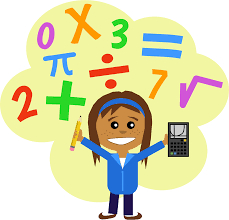• Save

# Maths questions series #2

3 years ago 121.8K Views## Very important Maths questions for SSC CGL-13 Series#2

21. Successive discount of 10% , 20% and 50% will be equivalent to a single discount of-

a. 36%
b. 64%
c. 80%
d. 56%

22. A retailer offers the following discount schemes for buyers on an article-

I. Two successive discounts of 10%
II. A discount of 12% followed by a discount of 8%.
III. Successive discounts of 15% and 5%
IV. A discount of 20%

The selling price will be minimum under the scheme-

a. I
b. II
c. III
d. IV

23. Of three numbers, the second is thrice the first and the third number is three-fourth of the first. If the average of the three numbers is 114, the largest number is –

a. 72
b. 216
c. 354
d. 726

24. A car covers 1/5 of the distance from A to B at the speed of 8 km/hour, 1/10 of the distance at 25 km per hour and the remaining at the speed of 20 km per hour. Find the average speed of the whole journey-

a. 12.625 km/hr
b. 13.625 km/hr
c. 14.625 km/hr
d. 15.625 km/hr

25. The average of 3 numbers is 154. The first number is twice the second and the second number is twice the third. The first number is-

a. 264
b. 132
c. 88
d. 66

26. The average salary of all the staff in an office of a corporate house is Rs. 5,000. The average salary of the officers is Rs. 14,000 and that of the rest is Rs. 4,000. If the total number of staff is 500, the number of officers is –

a. 10
b. 15
c. 25
d. 50

27. The average marks of 40 students in an English exam are 72. Later it is found that three marks 64, 62 and 84 were wrongly entered as 60, 65 and 73. The average after mistakes were rectified is-

a. 70
b. 72
c. 71.9
d. 72.1

28. A and B are two alloys of gold and copper prepared by mixing metals in the ratio 7:2 and 7:11 respectively. If equal quantities of the alloys are melted to form a third alloy C, the ratio of gold and copper in C will be-

a. 5 : 7
b. 5 : 9
c. 7 : 5
d. 9 : 5

29. In a laboratory, two bottles contain mixture of acid and water in the ratio 2 : 5 in the first bottle and 7 : 3 in the second. The ratio in which the contents of these two bottles be mixed such that the new mixture has acid and water in the ratio 2 : 3 is-

a. 4 : 15
b. 9 : 8
c. 21 : 8
d. 1 : 2

30. A mixture contains 80% acid and rest water. Part of the mixture that should be removed and replaced by same amount of water to make the ratio of acid and water 4 : 3 is-

a. 1/3 rd
b. 3/7 th
c. 2/3 rd
d. 2/7 th